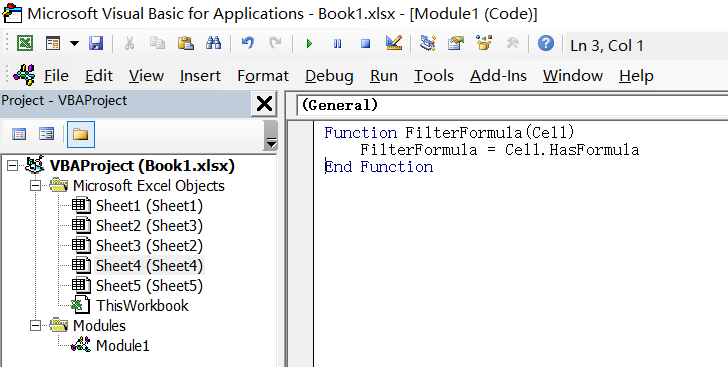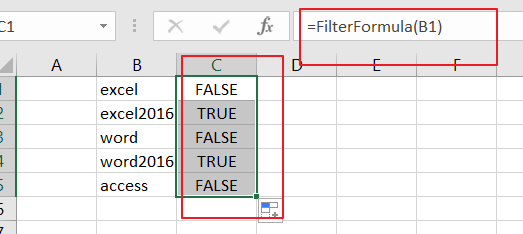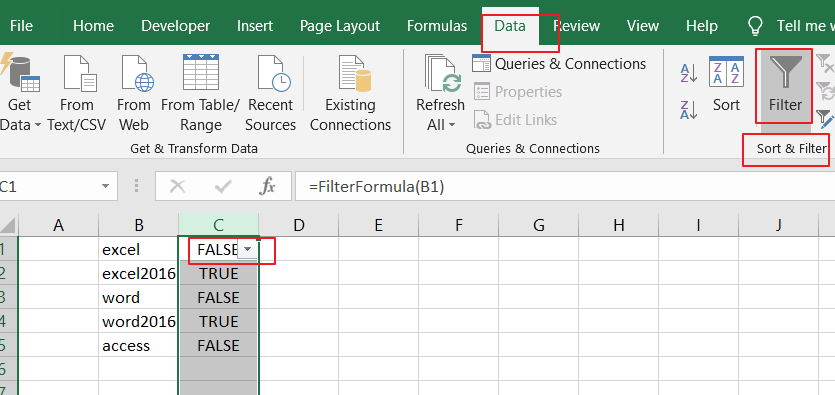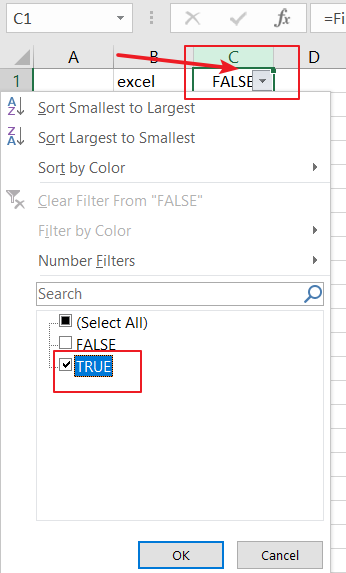# How to Filter Formula in Excel

This post will guide you how to filter your data with an Formula in Excel. How do I filter cells with formulas through a User defined function in Excel 2013/2016.

## Filter Formula

Assuming that you have a list of data in range B1:B5, in which contain some text values and formulas. and you want only to filter all formula cells in your worksheet. How to do it. The below steps will show you how to use a User Defined Function to filter Excel Formula.

Step1# open your excel workbook and then click on “Visual Basic” command under DEVELOPER Tab, or just press “ALT+F11” shortcut.Step2#  then the “Visual Basic Editor” window will appear.

Step3# click “Insert” ->”Module” to create a new module.Step4# paste the below VBA code into the code window. Then clicking “Save” button.```Function FilterFormula(Cell)
FilterFormula = Cell.HasFormula
End Function
```

Step5# back to the current worksheet, then type the following formula in a blank cell in helper column, and then press Enter key. And drag the AutoFill Handle over to other cells.

=FilterFormula(B1)Note: the above formula will check if your data contain Formula or not. If the formula returns True value, it indicates that this cell contain formula, otherwise, it just only a text value.

Step6# then you need to select your helper column, and go to Data tab, click Filter command under Sort & Filter group. And a Filter icon will add into the first cell in your helper column.Step7#  click the dropdown arrow in Cell C1 in your helper column, and only check TRUE option under the Select All section. And it will filter out all cells with formula.